# Test: Representation On Number Line

## 10 Questions MCQ Test Mathematics (Maths) Class 9 | Test: Representation On Number Line

Description
This mock test of Test: Representation On Number Line for Class 9 helps you for every Class 9 entrance exam. This contains 10 Multiple Choice Questions for Class 9 Test: Representation On Number Line (mcq) to study with solutions a complete question bank. The solved questions answers in this Test: Representation On Number Line quiz give you a good mix of easy questions and tough questions. Class 9 students definitely take this Test: Representation On Number Line exercise for a better result in the exam. You can find other Test: Representation On Number Line extra questions, long questions & short questions for Class 9 on EduRev as well by searching above.
QUESTION: 1

###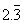is equivalent to the following on the number line.

Solution:

Let x = 2.333 (1) multiply both side with 10 = 10x = 23.333

(2) Now eq. (2) - (1) = 10x - x = 23.333 - 2.333 = 9x = 21 = x = 21/ 9 =x = 7/3

QUESTION: 2

Solution:
QUESTION: 3

### To represent √3 which of the following method would be used.

Solution:
QUESTION: 4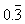is equivalent to the following on the number line.

Solution:
QUESTION: 5

To represent 9.3234 which of the following method would be used.

Solution:
QUESTION: 6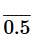is equivalent to the following on the number line.

Solution:
QUESTION: 7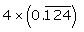is represented by the fraction_____________ on the number line.

Solution:
QUESTION: 8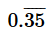lies between

Solution:
QUESTION: 9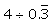is equivalent to the following on the number line

Solution:

option ( c) 12 is the correct answer.

Explanation:-

Let x = 0.333 ……… (1)  (now multiply both the sides with 10)

=> 10x = 3.333 ……(2)    now eq. 2 - eq.1

=> 10x - x = 3.333 - 0.333 => 9x = 3 => x = 3/9 => x = ⅓

Alq 4 ÷ ⅓  = 4 x 3 = 12

QUESTION: 10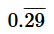is equivalent to the following on the number line

Solution: